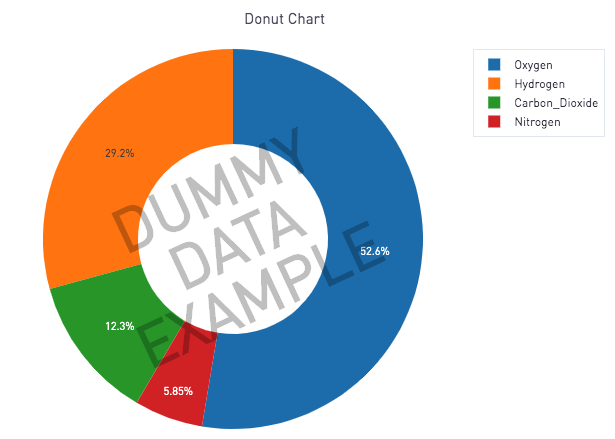Donut Charts — Plot.lyThe above example is taken from plot.ly's documentation here. A few updates have been made to the example to make it easier to use within the Periscope Python/R integration

Pie charts with a hole in it give us donut charts. Now many in the data visualization community have strong feelings about the usefulness (or lack thereof) of pie/donut charts as it is harder for the brain to pick up on differences in magnitude with a curve. However, sometimes it may be appropriate to use a donut chart. We welcome the debate in the comments below.

For those who are on team donut charts, please find the Python 3.6 code below. The code requires the SQL output to have the following 2 columns:

1. labels: the names of each "slice" in the donut chart
2. values: the values associated with each slice

If you don't have the above 2 columns in the correct format, you'll get a donut chart with dummy data as shown in the image above.

# Source: https://plot.ly/python/pie-charts/

# PERISCOPE DONUT CHART TEMPLATE
# SQL output should have 2 columns:
#    1) labels: the names of each "slice" in the donut chart
#    2) values: the values associated with each slice

# Import libraries
import pandas as pd
import plotly.plotly as py
import plotly.graph_objs as go

# Create Dummy Data
labels = ['Oxygen','Hydrogen','Carbon_Dioxide','Nitrogen']
values = [4500, 2500, 1053, 500]
data = {'labels':labels, 'values':values}
dummy_df = pd.DataFrame(data)
community_post = ''

# HELPER FUNCTION: For annotation text
style = ';'.join([f'{key.replace("_","-")}:{settings[key]}' for key in settings])
return f'<a href="{link}" style="{style}">{text}</a>'

# MAIN FUNCTION: input a dataframe
def plot(df, annotation = None):
# Use hole to create a donut-like pie chart
data = [
go.Pie(labels=df['labels'],
values=df['values'],
hole=.5)
]

layout = go.Layout(
title='Donut Chart',
margin=dict(
l=50,
r=50,
b=50,
t=50
),
annotations=[annotation]
)

fig = go.Figure(data = data, layout = layout)
periscope.plotly(fig)

# We try to to plot the SQL output. If it is not in the correct format, the dummy data will display with a watermark. See stdout tab for the error message.
try:
plot(df)
except Exception as e:
print(e)
annotation = {
'x': .5,
'y': .5,
'ax': 0,
'ay': 0,
'xref': 'paper',
'yref': 'paper',
'text': style_link('DUMMY<br><br><br><br>DATA<br><br><br><br>EXAMPLE', community_post, font_size='60px', font_weight='bold', color='rgba(0, 0, 0, .25)'),
'showarrow': False,
'textangle': -25
}
plot(dummy_df, annotation=annotation)